#### WATCH ALL SLIDES

Slide 1DO NOW: SOLVE THE INEQUALITY BY FOLLOWING THE STEPS WE HAVE LEARNED OVER THE LAST TWO DAYS. THEN WE WILL GRAPH TOGETHER ON THE CALCULATOR;

Slide 2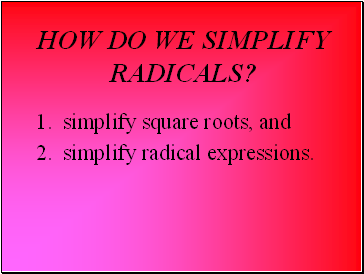## How do we simplify radicals?

1. simplify square roots, and

Slide 3In the expression , is the radical sign and 64 is the radicand.

If x2 = y then x is a square root of y.

1. Find the square root:

8

2. Find the square root:

-0.2

Slide 4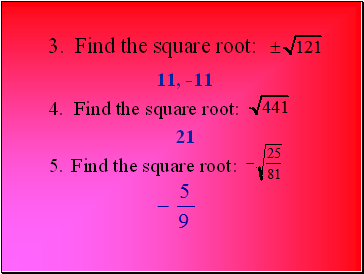11, -11

4. Find the square root:

21

5. Find the square root:

3. Find the square root:

Slide 56.82, -6.82

6. Use a calculator to find each square root. Round the decimal answer to the nearest hundredth.

Slide 61 • 1 = 1

2 • 2 = 4

3 • 3 = 9

4 • 4 = 16

5 • 5 = 25

6 • 6 = 36

49, 64, 81, 100, 121, 144, .

What numbers are perfect squares?

Slide 7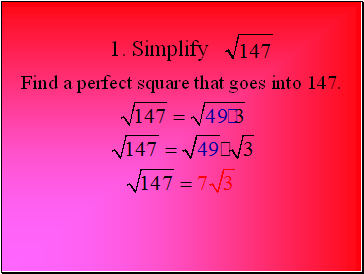1. Simplify

Find a perfect square that goes into 147.

Slide 82. Simplify

Find a perfect square that goes into 605.

Slide 9Simplify

.

.

.

.

Slide 10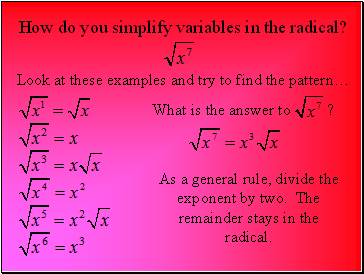Look at these examples and try to find the pattern…

How do you simplify variables in the radical?

What is the answer to ?

As a general rule, divide the exponent by two. The remainder stays in the radical.

Slide 11Find a perfect square that goes into 49.

4. Simplify

5. Simplify

Slide 12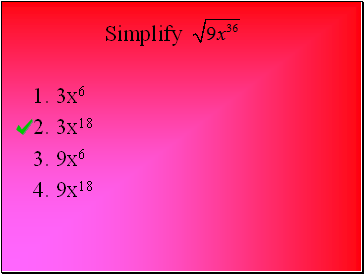Simplify

3x6

3x18

9x6

9x18

Slide 13What are Perfect Cubes?

13 = 1 x 1 x 1 = 1

23 = 2 x 2 x 2 = 8

33 = 3 x 3 x 3 = 27

43 = 4 x 4 x 4 = 64

53 = 5 x 5 x 5 = 125

and so on and on and on…

Slide 142

2

2 x 2 = 4

Slide 15Go to page:
1  2